21.2
21.1
20.2
20.1
19.2
19.1
The page you are viewing does not exist in version 19.1. This link will take you to the root page.
18.2
The page you are viewing does not exist in version 18.2. This link will take you to the root page.
18.1
The page you are viewing does not exist in version 18.1. This link will take you to the root page.
17.2
The page you are viewing does not exist in version 17.2. This link will take you to the root page.

w Function - Window Calculations at the Intermediate Aggregation Level

Overview

The w function uses window calculations to compute values for data rows within a window defined by specified criteria. You can use the function result as a measure to calculate summary values in a custom manner. You can wrap the function in an aggregate expression to use it as a dimension to group, sort, or filter data.

The demo uses the w function to highlight the maximum difference between product sales for a specific period:

The w function has the following syntax:

w(WindowExpression, partitionByFunction, orderByFunction)

You should specify all three parameters to set the w function.

Parameter Description Example
WindowExpression An aggregate expression or a one of the window calculation functions. RankDense(Sum([ProductSales]))
partitionBy(column1, column2, ...) A function that specifies the columns by which the data rows are grouped. The window function is applied to each partition separately. A parameter cannot be an aggregate expression. The partitionBy function can be used only as the w function argument. partitionBy([Product])
orderBy(column1, column2, ...) A function that specifies the calculation order for the window function. The data is calculated by the first parameter, then by the second and so on. You can wrap a parameter in the asc or desc function to change the calculation order. The orderBy function can be used only as the w function argument. orderBy(GetYear([Date]), desc(Sum([Sales])))

The table below describes additional functions used as the orderBy parameters:

Function Description Example
asc(column) Specifies the ascending calculation order for the specified column. This is the default calculation order and you can omit it to simplify an expression. The asc function can be used only as the orderBy function argument. w(Index(), partitionBy(), orderBy(asc(Sum([Sales]))))
desc(column) Specifies the descending calculation order for the specified column. The desc function can be used only as the orderBy function argument. w(Index(), partitionBy(), orderBy(desc(Sum([Sales]))))

Note

The w function is available only for client data processing mode.

Partition

The following example shows how the partition works. The grid below has three columns (Year, Month, and Count) and displays the products aggregated by months and years: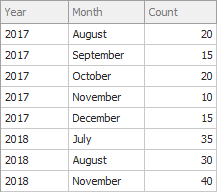Create a calculated field with the following expression that contains the w function:

w(RunningSum(Sum([Count])), partitionBy([Year]), orderBy([Month]))

The added calculated field displays the running totals for each year. The partitionBy function defines a year as a partition: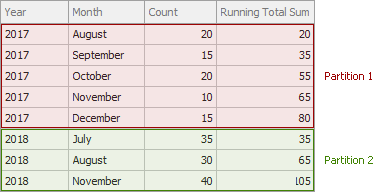Add one more partition to calculate running totals first by year and then by quarters:

w(RunningSum(Sum([Count])), partitionBy(GetYear([Date]), GetQuarter([Date])), orderBy(GetMonth([Date])))

The resulting expression calculates running totals in four partitions separately: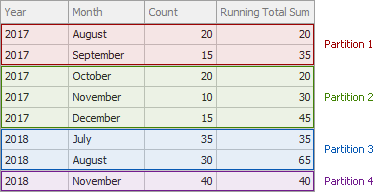Tables below show examples of valid and invalid expressions:

Valid

Expression Description
paritionBy() Empty parameters define a partition as a whole set of rows.
partitionBy([Category], [Product]) The expression specifies a partition by products in each category.
partitionBy(aggr(Sum(x), y, z)) A complex partition definition that contains a non-aggregate expression.

Invalid

Expression Description
partitionBy(Sum([Sales])) A parameter cannot be an aggregate expression.
partitionBy(GetYear(Min([Date])) - 2000) A complex aggregate expression.
partitionBy([Category], Sum([Sales])) One of the parameters is an aggregate expression.

Order

The orderBy function specifies the calculation order for the window function. The parameters can be a list or empty set of expressions that define the calculation order inside a partition.

You can add the asc or desc function to revert the calculation order. For example, create a calculated field with the following expression:

w(RunningSum(Sum([Count])), partitionBy([Year]), orderBy([Month]))

The default order is ascending. In this case, the expression calculates the running totals from the first to the last month: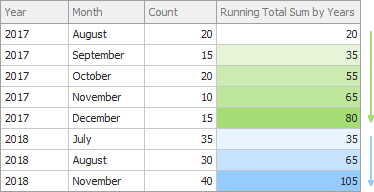To revert the calculation order, wrap the expression inside orderBy in the desc function:

w(RunningSum(Sum([Count])), partitionBy([Year]), orderBy(desc([Month])))

As a result, the running totals are calculated from the last to the first month: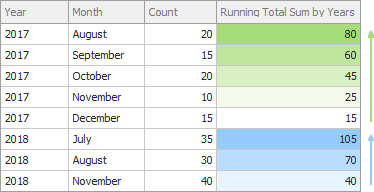A tables below shows examples of valid expressions:

Valid

Expression Description
orderBy() Empty parameters do not specify the calculation order.
orderBy(Sum([Sales)) The parameter specifies the ascending order and sorts values by the Sum[Sales] aggregate expression.
orderBy(asc(Sum([Sales]))) The parameter specifies the ascending order and sorts values by the Sum[Sales] aggregate expression. You can omit asc to simplify an expression.
orderBy(desc(Sum([Sales]))) The parameter specifies the descending order and sorts values by the Sum[Sales] aggregate expression.
orderBy(GetYear(Date)) The parameter specifies the descending order and sorts values by the GetYear(Date) non-aggregate expression.

Example - How to Filter Data and Display Top N Values

The example below shows how to filter data to display top N products in each category in the WinForms Dashboard Designer.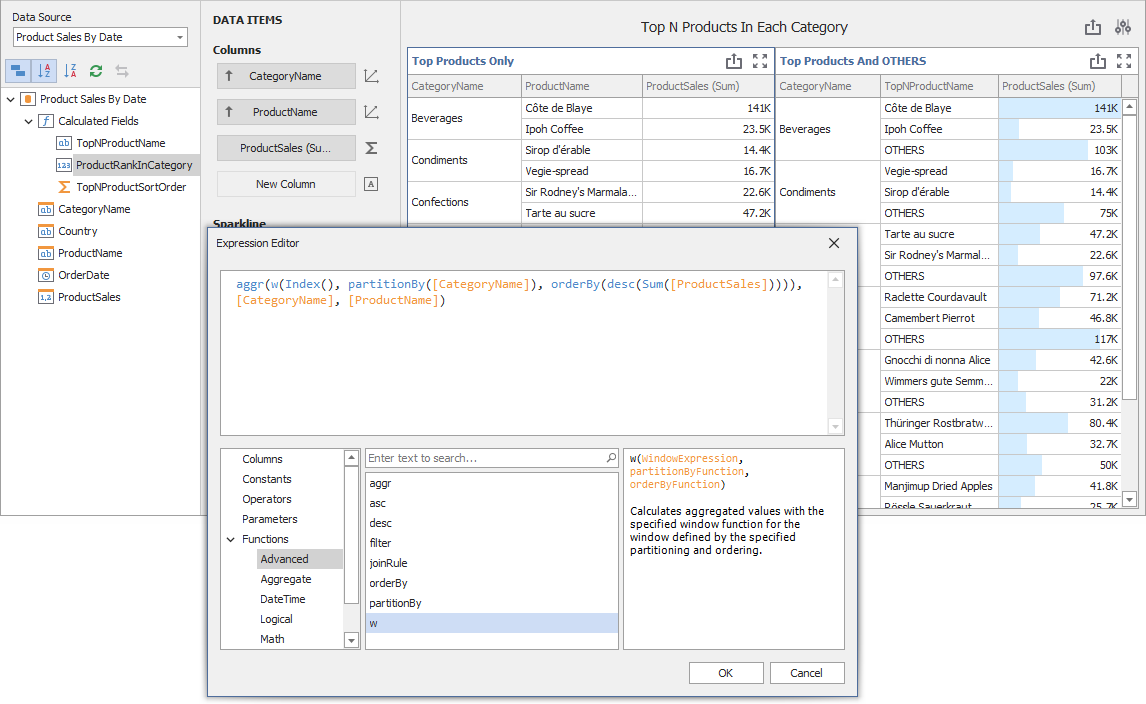The ProductRankInCategory calculated field contains the following expression:

aggr(w(Index(), partitionBy([CategoryName]), orderBy(desc(Sum([ProductSales])))), [CategoryName], [ProductName])
• The expression divides data rows into partitions according to CategoryName, gets the index of the row in each partition, and sorts ProductSales totals in descending order.
• The CategoryName and ProductName fields specify that the aggr function aggregates data for each category and product.
• The first parameter of the aggregation is the w function expression. This is a window function that calculates index in a window.

The grid displays the Top N records (TopValueCount parameter) of the ProductRankInCategory expression’s result, and combines the remaining records into the OTHER value:

Iif([ProductRankInCategory] <= ?TopValueCount, [ProductName], 'OTHERS')

You can change the TopValueCount parameter to specify how many values to display as Top N.